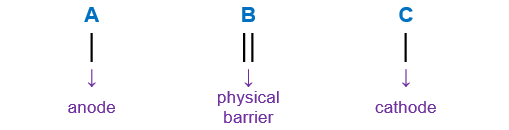# Problem: For the following electrochemical cellCu(s) | Cu2+(aq,0.0155M) || Ag+(aq,1.50M | Ag(s)Write the net cell equation. Phases are optional.Calculate the following values at 25.0°C using standard potentials as needed.E*cell = VEcell = VΔG°rxn = kJ/molΔGrxn = kJ/mol

###### FREE Expert Solution

Cu(s) | Cu2+(aq,0.0155M) || Ag+(aq,1.50M | Ag(s)

When writing a cell notation, we use the following format – “as easy as ABCLose               Gain
Electron         Electrons
Oxidation       Reduction

lose electrons oxidation → anode
gain electrons reduction → cathode

91% (156 ratings)###### Problem Details

For the following electrochemical cell

Cu(s) | Cu2+(aq,0.0155M) || Ag+(aq,1.50M | Ag(s)

Write the net cell equation. Phases are optional.

Calculate the following values at 25.0°C using standard potentials as needed.

E*cell = V

Ecell = V

ΔG°rxn = kJ/mol

ΔGrxn = kJ/mol